# Selina Solutions Concise Maths Class 10 Chapter 8 Remainder and Factor Theorems Exercise 8(B)

Using the factor theorem to factorise the given polynomial is the main concept discussed in this exercise. The students wishing to strengthen their problem-solving abilities on this chapter and others can use the Selina Solutions for Class 10 Maths. Experts at BYJU’S have created this valuable resource in order to help students prepare confidently for their exams. The solutions to the Concise Selina Solutions for Class 10 Maths Chapter 8 Remainder and Factor Theorems Exercise 8(B) are available in PDF format, given in the links given below.

## Selina Solutions Concise Maths Class 10 Chapter 8 Remainder and Factor Theorems Exercise 8(B) Download PDF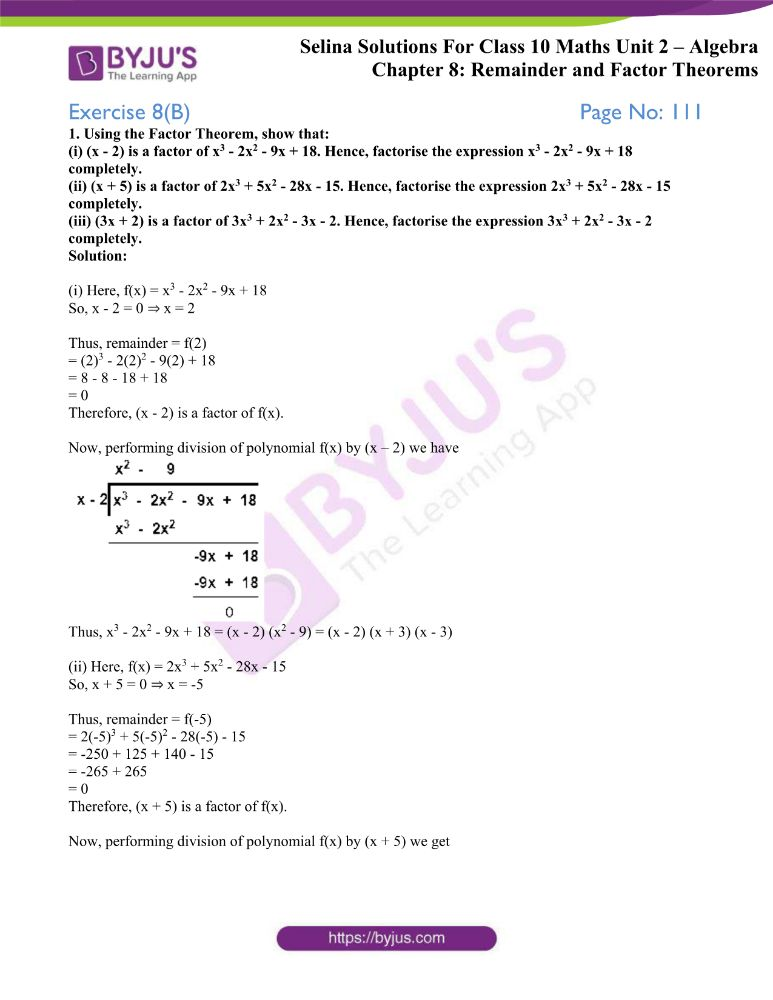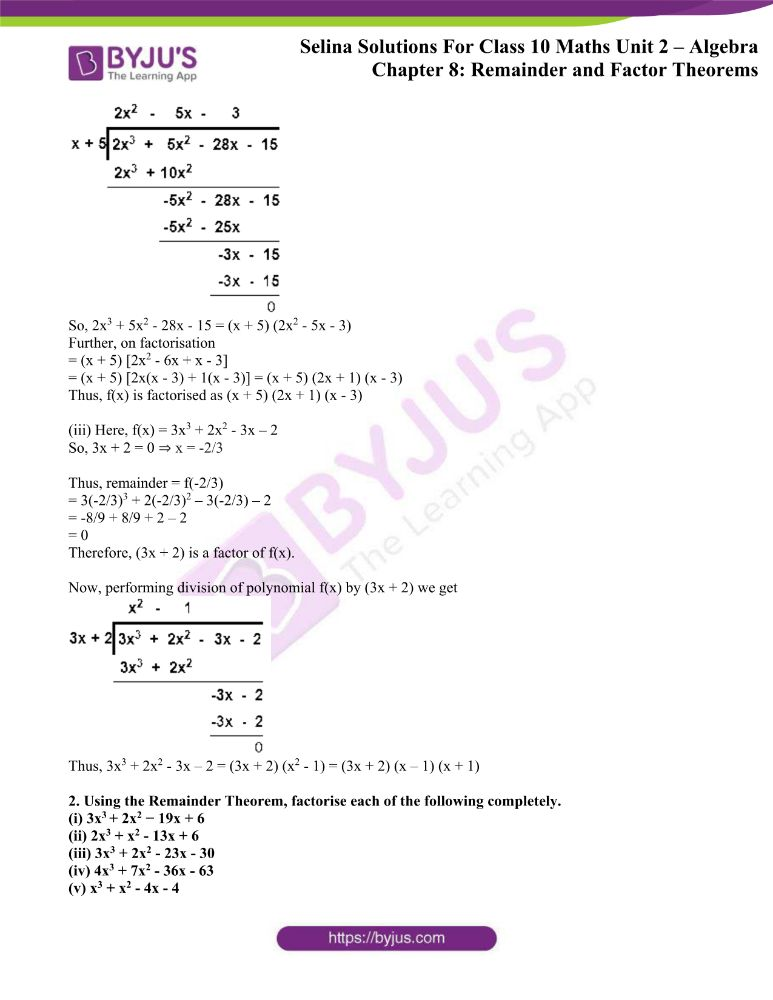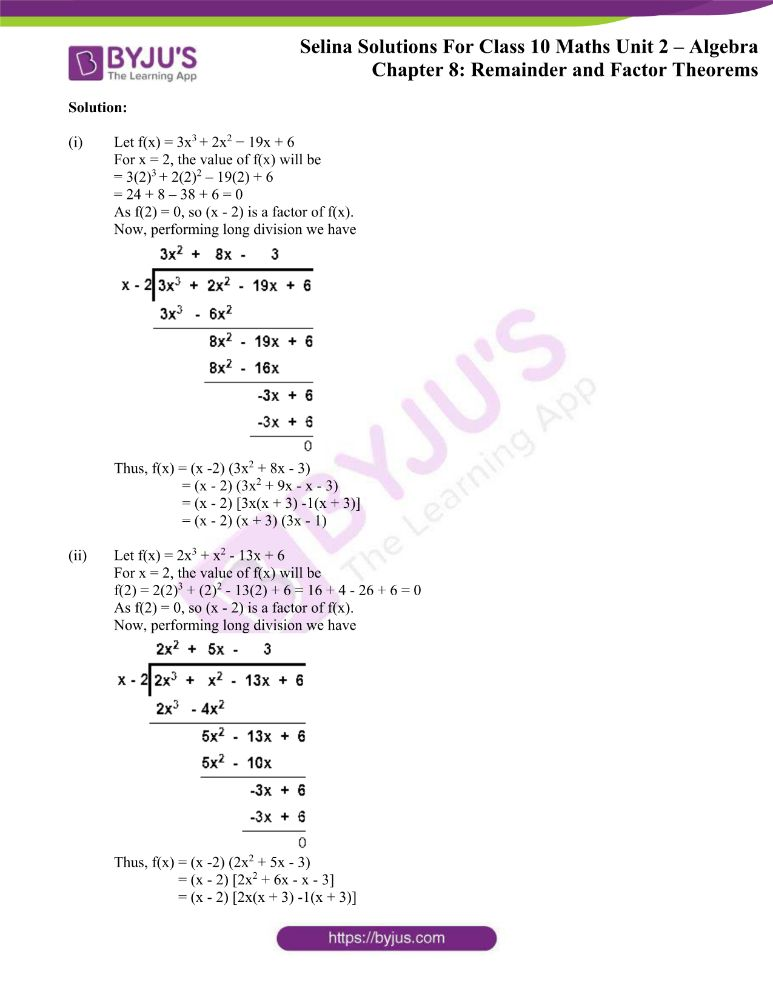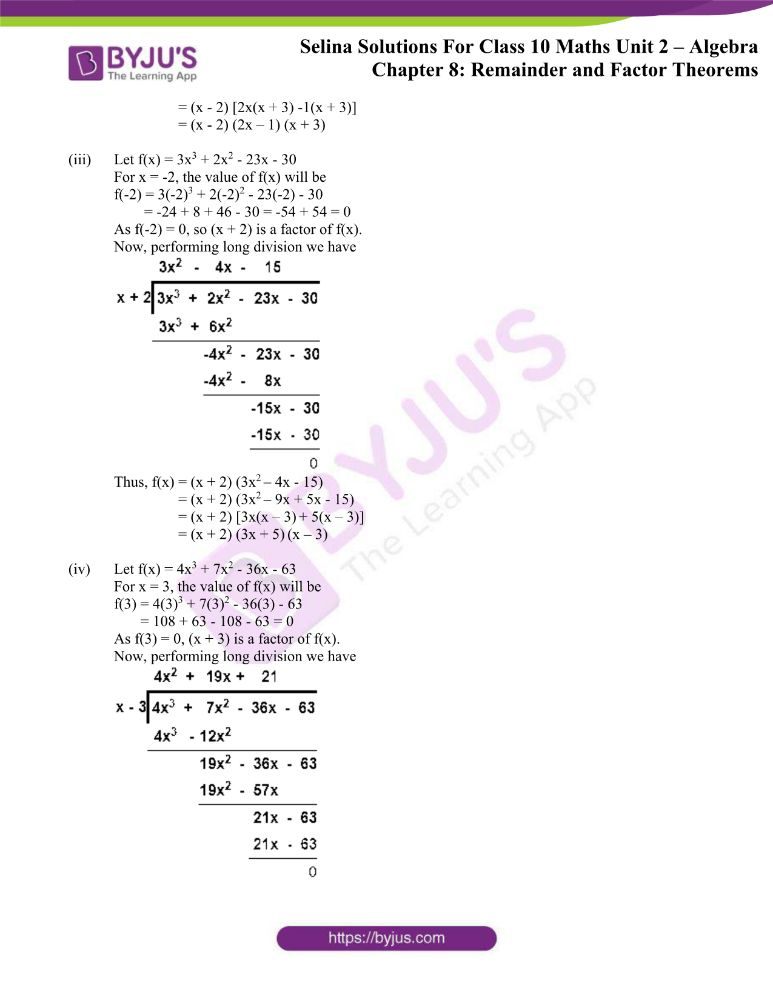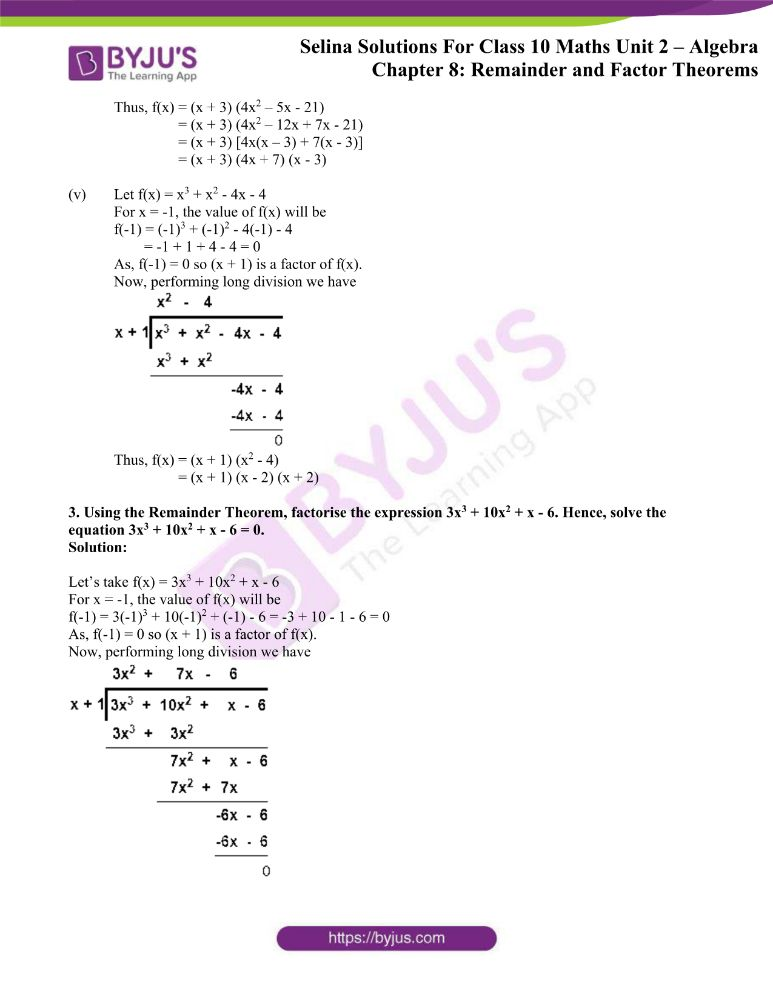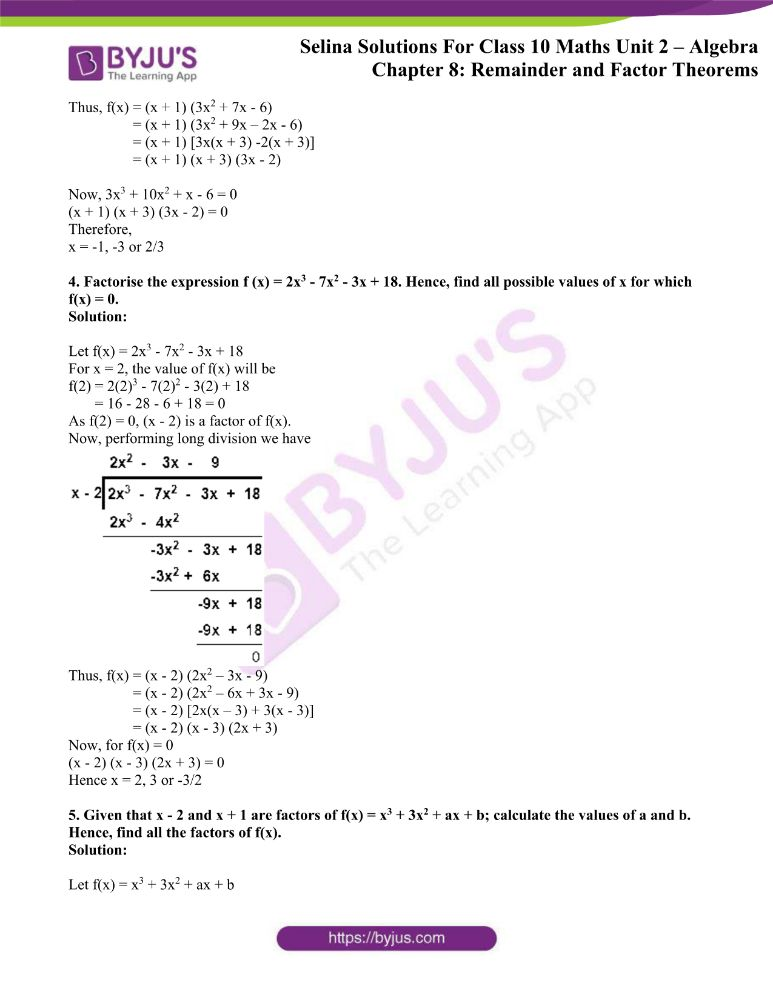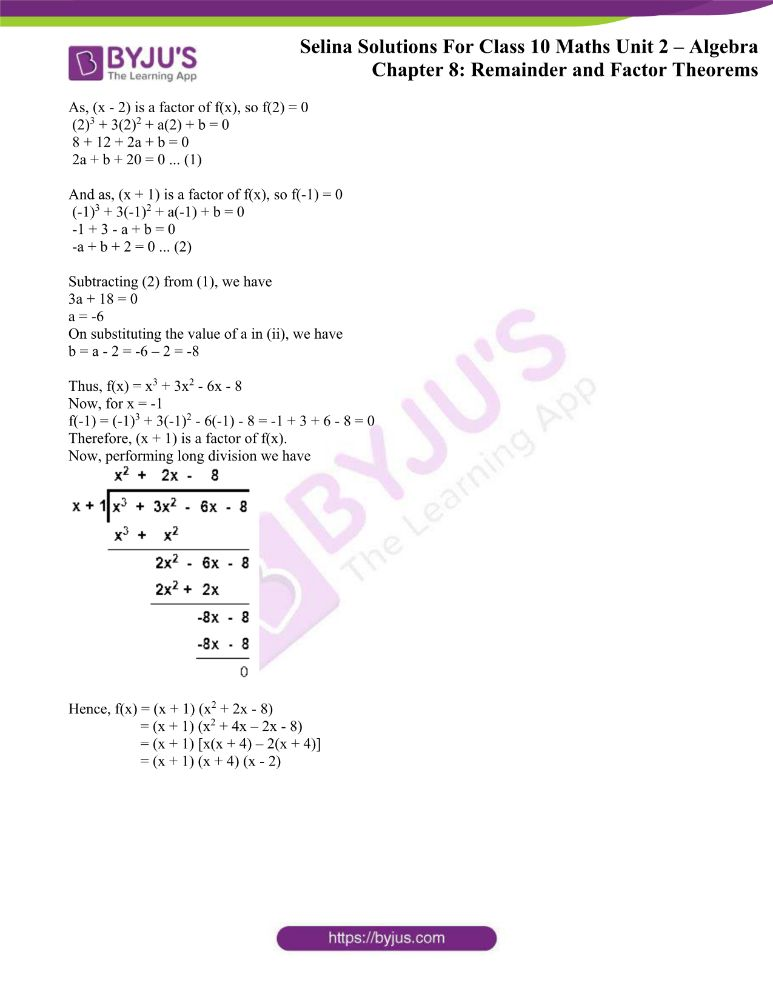### Access other exercises of Selina Solutions Concise Maths Class 10 Chapter 8 Remainder and Factor Theorems

Exercise 8(A) Solutions

Exercise 8(C) Solutions

### Access Selina Solutions Concise Maths Class 10 Chapter 8 Remainder and Factor Theorems Exercise 8(B)

1. Using the Factor Theorem, show that:

(i) (x – 2) is a factor of x3 – 2x2 – 9x + 18. Hence, factorise the expression x3 – 2x2 – 9x + 18 completely.

(ii) (x + 5) is a factor of 2x3 + 5x2 – 28x – 15. Hence, factorise the expression 2x3 + 5x2 – 28x – 15 completely.

(iii) (3x + 2) is a factor of 3x3 + 2x2 – 3x – 2. Hence, factorise the expression 3x3 + 2x2 – 3x – 2 completely.

Solution:

(i) Here, f(x) = x3 – 2x2 – 9x + 18

So, x – 2 = 0 ⇒ x = 2

Thus, remainder = f(2)

= (2)3 – 2(2)2 – 9(2) + 18

= 8 – 8 – 18 + 18

= 0

Therefore, (x – 2) is a factor of f(x).

Now, performing division of polynomial f(x) by (x – 2) we have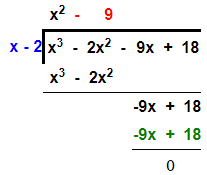Thus, x3 – 2x2 – 9x + 18 = (x – 2) (x2 – 9) = (x – 2) (x + 3) (x – 3)

(ii) Here, f(x) = 2x3 + 5x2 – 28x – 15

So, x + 5 = 0 ⇒ x = -5

Thus, remainder = f(-5)

= 2(-5)3 + 5(-5)2 – 28(-5) – 15

= -250 + 125 + 140 – 15

= -265 + 265

= 0

Therefore, (x + 5) is a factor of f(x).

Now, performing division of polynomial f(x) by (x + 5) we get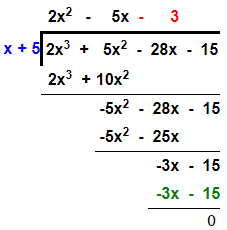So, 2x3 + 5x2 – 28x – 15 = (x + 5) (2x2 – 5x – 3)

Further, on factorisation

= (x + 5) [2x2 – 6x + x – 3]

= (x + 5) [2x(x – 3) + 1(x – 3)] = (x + 5) (2x + 1) (x – 3)

Thus, f(x) is factorised as (x + 5) (2x + 1) (x – 3)

(iii) Here, f(x) = 3x3 + 2x2 – 3x – 2

So, 3x + 2 = 0 ⇒ x = -2/3

Thus, remainder = f(-2/3)

= 3(-2/3)3 + 2(-2/3)2 – 3(-2/3) – 2

= -8/9 + 8/9 + 2 – 2

= 0

Therefore, (3x + 2) is a factor of f(x).

Now, performing division of polynomial f(x) by (3x + 2) we get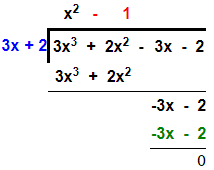Thus, 3x3 + 2x2 – 3x – 2 = (3x + 2) (x2 – 1) = (3x + 2) (x – 1) (x + 1)

2. Using the Remainder Theorem, factorise each of the following completely.

(i) 3x+ 2x2 − 19x + 6

(ii) 2x3 + x2 – 13x + 6

(iii) 3x3 + 2x2 – 23x – 30

(iv) 4x3 + 7x2 – 36x – 63

(v) x3 + x2 – 4x – 4

Solution:

(i) Let f(x) = 3x+ 2x2 − 19x + 6

For x = 2, the value of f(x) will be

= 3(2)+ 2(2)2 – 19(2) + 6

= 24 + 8 – 38 + 6 = 0

As f(2) = 0, so (x – 2) is a factor of f(x).

Now, performing long division we have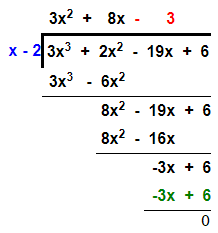Thus, f(x) = (x -2) (3x2 + 8x – 3)

= (x – 2) (3x2 + 9x – x – 3)

= (x – 2) [3x(x + 3) -1(x + 3)]

= (x – 2) (x + 3) (3x – 1)

(ii) Let f(x) = 2x3 + x2 – 13x + 6

For x = 2, the value of f(x) will be

f(2) = 2(2)3 + (2)2 – 13(2) + 6 = 16 + 4 – 26 + 6 = 0

As f(2) = 0, so (x – 2) is a factor of f(x).

Now, performing long division we have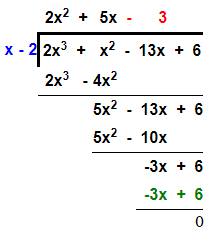Thus, f(x) = (x -2) (2x2 + 5x – 3)

= (x – 2) [2x2 + 6x – x – 3]

= (x – 2) [2x(x + 3) -1(x + 3)]

= (x – 2) [2x(x + 3) -1(x + 3)]

= (x – 2) (2x – 1) (x + 3)

(iii) Let f(x) = 3x3 + 2x2 – 23x – 30

For x = -2, the value of f(x) will be

f(-2) = 3(-2)3 + 2(-2)2 – 23(-2) – 30

= -24 + 8 + 46 – 30 = -54 + 54 = 0

As f(-2) = 0, so (x + 2) is a factor of f(x).

Now, performing long division we have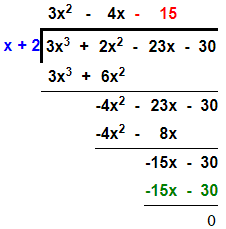Thus, f(x) = (x + 2) (3x2 – 4x – 15)

= (x + 2) (3x2 – 9x + 5x – 15)

= (x + 2) [3x(x – 3) + 5(x – 3)]

= (x + 2) (3x + 5) (x – 3)

(iv) Let f(x) = 4x3 + 7x2 – 36x – 63

For x = 3, the value of f(x) will be

f(3) = 4(3)3 + 7(3)2 – 36(3) – 63

= 108 + 63 – 108 – 63 = 0

As f(3) = 0, (x + 3) is a factor of f(x).

Now, performing long division we have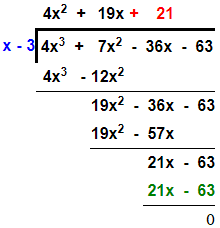Thus, f(x) = (x + 3) (4x2 – 5x – 21)

= (x + 3) (4x2 – 12x + 7x – 21)

= (x + 3) [4x(x – 3) + 7(x – 3)]

= (x + 3) (4x + 7) (x – 3)

(v) Let f(x) = x3 + x2 – 4x – 4

For x = -1, the value of f(x) will be

f(-1) = (-1)3 + (-1)2 – 4(-1) – 4

= -1 + 1 + 4 – 4 = 0

As, f(-1) = 0 so (x + 1) is a factor of f(x).

Now, performing long division we have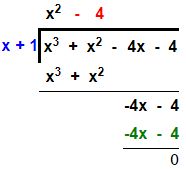Thus, f(x) = (x + 1) (x2 – 4)

= (x + 1) (x – 2) (x + 2)

3. Using the Remainder Theorem, factorise the expression 3x3 + 10x2 + x – 6. Hence, solve the equation 3x3 + 10x2 + x – 6 = 0.

Solution:

Let’s take f(x) = 3x3 + 10x2 + x – 6

For x = -1, the value of f(x) will be

f(-1) = 3(-1)3 + 10(-1)2 + (-1) – 6 = -3 + 10 – 1 – 6 = 0

As, f(-1) = 0 so (x + 1) is a factor of f(x).

Now, performing long division we have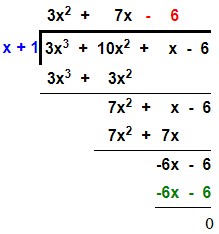Thus, f(x) = (x + 1) (3x2 + 7x – 6)

= (x + 1) (3x2 + 9x – 2x – 6)

= (x + 1) [3x(x + 3) -2(x + 3)]

= (x + 1) (x + 3) (3x – 2)

Now, 3x3 + 10x2 + x – 6 = 0

(x + 1) (x + 3) (3x – 2) = 0

Therefore,

x = -1, -3 or 2/3

4. Factorise the expression f (x) = 2x3 – 7x2 – 3x + 18. Hence, find all possible values of x for which f(x) = 0.

Solution:

Let f(x) = 2x3 – 7x2 – 3x + 18

For x = 2, the value of f(x) will be

f(2) = 2(2)3 – 7(2)2 – 3(2) + 18

= 16 – 28 – 6 + 18 = 0

As f(2) = 0, (x – 2) is a factor of f(x).

Now, performing long division we have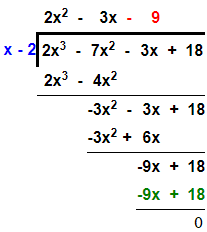Thus, f(x) = (x – 2) (2x2 – 3x – 9)

= (x – 2) (2x2 – 6x + 3x – 9)

= (x – 2) [2x(x – 3) + 3(x – 3)]

= (x – 2) (x – 3) (2x + 3)

Now, for f(x) = 0

(x – 2) (x – 3) (2x + 3) = 0

Hence x = 2, 3 or -3/2

5. Given that x – 2 and x + 1 are factors of f(x) = x3 + 3x2 + ax + b; calculate the values of a and b. Hence, find all the factors of f(x).

Solution:

Let f(x) = x3 + 3x2 + ax + b

As, (x – 2) is a factor of f(x), so f(2) = 0

(2)3 + 3(2)2 + a(2) + b = 0

8 + 12 + 2a + b = 0

2a + b + 20 = 0 … (1)

And as, (x + 1) is a factor of f(x), so f(-1) = 0

(-1)3 + 3(-1)2 + a(-1) + b = 0

-1 + 3 – a + b = 0

-a + b + 2 = 0 … (2)

Subtracting (2) from (1), we have

3a + 18 = 0

a = -6

On substituting the value of a in (ii), we have

b = a – 2 = -6 – 2 = -8

Thus, f(x) = x3 + 3x2 – 6x – 8

Now, for x = -1

f(-1) = (-1)3 + 3(-1)2 – 6(-1) – 8 = -1 + 3 + 6 – 8 = 0

Therefore, (x + 1) is a factor of f(x).

Now, performing long division we have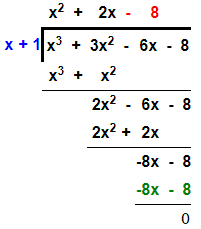Hence, f(x) = (x + 1) (x2 + 2x – 8)

= (x + 1) (x2 + 4x – 2x – 8)

= (x + 1) [x(x + 4) – 2(x + 4)]

= (x + 1) (x + 4) (x – 2)